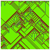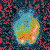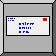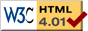# Perceptron Geometry

This page was automatically generated by NetLogo 4.1. Questions, problems? Contact feedback@ccl.northwestern.edu.

The applet requires Java 5 or higher. Java must be enabled in your browser settings. Mac users must have Mac OS X 10.4 or higher. Windows and Linux users may obtain the latest Java from Sun's Java site. If the display appear cut off with Firefox, then try another browser (Safari works).

## WHAT IS IT?

This model demonstrates the geometry of the Perceptron Learning Algorithm. It generates a linearly separable, or almost linearly separable, set of data, and shows how a weight vector can be adjusted so that a single perceptron is able to separate the positive and negative data points. Two classes of data are linearly separable if they can be separated by a straight line, flat plane, or a flat hyperplane (for dimensions greater than 3).

A perceptron is a simple artificial neuron with n inputs and one binary output. Each input k has a weight (synaptic strength) w_k, and so we can treat the n weights as a vector, w = (w_1, ..., w_n). Each neuron also has a threshold t, and if the weighted inputs are greater than the threshold, the neuron produces a 1 output, otherwise it produces a 0 output. Therefore, if the input is a vector x = (x_1, ..., x_n), then the output will be 1 if w_1 x_1 + ... + w_n x_n > t, and 0 otherwise. In vector notation, we can say the output is 1 if the dot product w.x > t.

Suppose we are given a dataset comprising a number p of data vectors x1, x2, ..., xp, and that some of these are positive examples of some class of patterns (i.e., they fit the pattern), and others are negative examples (they don't fit). The perceptron learning problem is to find a single threshold and set of weights so that the perceptron correctly classifies all these data (and, hopefully, other data of a similar kind). The perceptron learning algorithm solves this problem.

There are several things we can do to simplify perceptron learning. First, we can eliminate the threshold by treating it as an extra, "zeroth" weight. To do this, we add a corresponding zeroth element to the data vectors, which is always -1. In effect the zeroth input is "clamped" at a -1 value. To see why this works, define an extended weight vector W where W_0 = t, W_1 = w_1, ..., W_n = w_n. Also, let X be the "extended" vector corresponding to x: X = (-1, x_1, x_2, ..., x_n). So now we are working with n+1 dimensional vectors. Note that:

W.X = t (-1) + w_1 x_1 + ... + w_n x_n = w.x - t

Thus w.x > t if and only if W.X > 0. Therefore, since the threhold has been turned into a weight, we only have to worry about adjusting weights.

The second simplification is to find a way to treat the positive and negative examples the same way. If X is a positive example, we want W.X > 0, but if X is a negative example, we want W.X < 0. However, since W.(-X) = -W.X, we know that W.X < 0 if and only if W.(-X) > 0. Therefore, we can replace the negative examples by their complements and treat them like positive examples. Specifically, we will define a new set of test data Z1, ..., Zp corresponding to the original data X1, ..., Xp. If Xi is a positive sample, then Zi = Xi, but if Xi is a negative sample, then Zi = -Xi.

Therefore, we have a simplified perceptron training problem, which is to find a weight wector W such that W.Z > 0 for every (modified) sample data vector Z.

For ease in visualization, this model uses two-dimensional data and weight vectors. It generates random test data, linearly separable into positive and negative examples. The model then converts all the data to positive example, as explained above, and demonstrates the adjustment of the weight vector so that all the test data are on the same (positive) side of the separating line.

## HOW IT WORKS

The learning procedure is as follows. The algorithm selects a random data point. If it is already classified correctly (i.e., W.Z > 0), the algorithm does nothing. If it is classified incorrectly (W.Z <= 0), then it alters the weight vector (to W') so that the classification is closer to being correct (W'.Z > W.Z). It does this by vector addition of a fraction (eta) of the data vector to the weight vector in order to produce the new weight vector: W' = W + eta * Z. The model displays this vector addition, which can be seen most clearly by stepping through the update process (clicking repeatedly in order: Test One Datum, Calculate New Weight, and Update Weight).

Eta determines the learning rate, but if it is too high, it may lead to instability, since the weight vector may adapt too much to the most recent datum, and "forget" previous ones.

## HOW TO USE IT

NUM DATA POINTS determines the number of random test data points to be generated. They have an equal porbability of being positive (shown in red) or negative (shown in blue). The two classes are all or mostly linearly separable.

CORRECT SEPARATOR set the angle of the normal vector to the line through the origin that will separate the positive from the negative samples (except for the exceptions: see next).

NON-LINEAR-SEPARABLE determines the percentage of samples that are not linearly separable. They are located randomly and are randomly positive or negative. Set this slider to 0 in order to have the positive and negative samples separated by Correct Separator.

SETUP generates the requested number of sample data points, classified according to the specified separator and percentage of exception. Positive and negative samples are indicated by red and blue, respectively. The initial, randomly chosen weight vector is shown in green, along with the separating line perpendicular to it.

REFLECT NEGATIVES converts all the samples to positive samples by replacing the negative samples by their negations. After Setup, you should click Reflect Negatives so that all the data are on one side of the separator.

ETA is the learning rate. It can be changed while the model is running. For example decreasing Eta may allow a non-converging learning process to converge.

To walk through learning process step by step, use the following three buttons:

TEST ONE DATUM picks a random data point for learning; it is indicated by yellow color.

CALCULATE NEW WEIGHT calculates the new weight vector (blue), which is shown as a vector sum of the old weight vector (green) and Eta times the test datum (yellow).

UPDATE WEIGHT replaces the old weight vector by the new one, and rotates the separating line accordingly.

The following controls allow the learning algorithm to be run continuously:

DELAY is the amount of time between each of the above three steps in continuous update (GO) mode. You can change the Delay while the model is running in order to speed it up or slow it down.

GO continuously cycles through the three steps, Test One Datum, Calculate New Weight, and Update Weight.

LEARNED ANGLE displays the angle of the learned weight vector, which may be compared to the angle set by Correct Separator.

## THINGS TO NOTICE

Notice that if the learning rate Eta is too high, the learning process may not converge. Try decreasing Eta to see if it converges.

Observe the differences depending on whether the initial random weight vector tends to point in about the same direction as the positive test, or tends to point in the opposite direction.

Observe the effects of non-linearly separable data on learning convergence.

Notice how close the angle of the learned weight vector comes to approximate the angle of the separator used to generate the test data. Can you think of ways of altering the learning algorithm to make the approximation closer?

## THINGS TO TRY

Try various learning rates (Eta). The Perceptron Learning Theorem proves that for linearly separable data the Perceptron Learning Algorithm will always converge *if the learning rate is sufficiently slow*. On the other hand, we would like learning to go as fast as possible. Run some experiments and determine a learning rate that always, or almost always, converges. Does it depend on the number of data points?

Try introducing a small percentage (e.g., 5%) of non-linearly separable data, and observe the behavior of the model with several randomly generated datasets.

## EXTENDING THE MODEL

Notice that the weight vector often gets shorter as it is updated, which could lead to numerical problems with a large number of updates. Since only the direction of the weight vector matters, how could you modify the model keep it from getting shorter?

Since all that really matters is the directions of the vectors, and not their lengths, consider modifying the algorithm to work in terms of normalized vectors. How does this new algorithm compare with the original one.

The original perceptron learning algorithm, embodied in this model, makes no weight adjustment for data that are classified correctly ("if it ain't broke, don't fix it"). Can you think of some useful adjustment that could be done for correctly classified data? Try out your idea.

## NETLOGO FEATURES

This model uses the links feature to draw the vectors and the left and right segments of the separating line. The endpoints of these lines are represented by turtles (sometimes hidden).

## RELATED MODELS

There are two related models in the standard Models Library (Computer Science, unverfied): Perceptron, which gives a neural network view of perceptron learning, and Artificial Neural Net, which demonstrates the back-propagation learning (essentially multilayer perceptron learning).

## CREDITS AND REFERENCES

The perceptron, which was one of the first artificial neural net models, was developed by Frank Rosenblatt in the late 1950s. It is described in most textbooks on artificial neural networks, but this particular model follows Ballard (1997, ¤8.2).

Rosenblatt, F. (1958). The perceptron: A probabilistic model for information storage and organization in the brain. Psychological Review, 65, 386-408.

Rosenblatt, F. (1962). Principles of neurodynamics. Spartan Books.

Ballard, D.H. (1997). An introduction to natural computation. MIT Press.

To refer to this model in academic publications, please use: MacLennan, B.J. (2008). NetLogo Perceptron Geometry model. http://www.cs.utk.edu/~mclennan. Dept. of Electrical Engineering & Computer Science, Univ. of Tennessee, Knoxville.

## PROCEDURES

```globals [ max-X ; X coordinates are +- max-Xmax-Y ; Y coordinates are +- max-Ytest-datum ; the current datum under testweight ; the current weight vectornew-weight ; temporary new weight vectorweight-change? ; set if a weight has changedlearned-angle ; the angle of the currrent weight vector]breed [ data datum ] ; type of the data pointsbreed [ origins origin ] ; type of the origin pointbreed [ weight-vectors weight-vector ] ; type of the weight vectordirected-link-breed [ normal-vectors normal-vector ] ; normal vector to separatorundirected-link-breed [ separatrices separatrix ] ; separator line segmentsbreed [ septx-ends septx-end ] ; end points of separatordirected-link-breed [ data-vectors data-vector ] ; visible data vectorsdata-own [ positive? ] ; set if it is a positive exampleseptx-ends-own [ theta ] ; angle separator ends (+- 90)to setup caset max-X max-pxcor - 1set max-Y max-pycor - 1create-origins 1 [ initialize-origin ] create-data num_data_points [ initialize-data ] create-weight-vectors 1 [ initialize-weight-vector ] create-septx-ends 1 [ initialize-separatrix 90 ] create-septx-ends 1 [ initialize-separatrix -90 ] set weight-change? falseset learned-angle [ atan xcor ycor ] of weightendto initialize-origin set size 2set shape "circle"set color greensetxy 0 0endto initialize-data set size 2set shape "circle"set color redset xcor (random (2 * max-X) - max-X) set ycor (random (2 * max-Y) - max-Y) ifelse non-linear-separable <= random 100 ; generate lin-sep data[ ifelse (xcor * sin correct_separator + ycor * cos correct_separator) > 0[ set positive? true ] [ set positive? false ] ] [ ifelse 0 = random 1 ; generate non-lin-sep data[ set positive? true ] [ set positive? false ] ] set color ifelse-value positive? [ red ] [ blue ]end;; Generate initial random weight vector;; Weight-vector procedureto initialize-weight-vector hide-turtlelet init-theta random 360let magnitude 0.9 * max-X setxy (magnitude * sin init-theta) (magnitude * cos init-theta) create-normal-vector-from origin 0 [ set thickness 0.5set color green]set weight selfend;; Generate separator line segments relative to weight vector;; Separatrix endpoint (septx-end) procedureto initialize-separatrix [init-theta] ; theta = +- 90hide-turtleset theta init-theta move-separatrix create-separatrix-with origin 0 [ set thickness 0.5set color green]end;; Reposition separatrix endpoint relative to weight vector;; Separatrix endpoint (septx-end) procedureto move-separatrix setxy 0 0set heading theta + towards weight fd max-Xend;; Convert negative examples to positive examplesto reflect-negatives let w_X [ xcor ] of weight let w_Y [ ycor ] of weight ask data [ if not positive? [ setxy (- xcor) (- ycor) ] ] wait 0.5 ; so that color change is visibleask data [ set color red ]end;; Cycle through learning procedure stepsto go test-one wait delay calculate-new-weight wait delay update-weight wait delayend;; Select random datum for testingto test-one set test-datum one-of data ask test-datum [ set color yellow  create-data-vector-from origin 0 [ set color yellow]]set weight-change? falseend;; Calculate new weight vector (if necessary) for test datumto calculate-new-weight let old_X [ xcor ] of weight let old_Y [ ycor ] of weight let datum_X [ xcor ] of test-datum let datum_Y [ ycor ] of test-datum if (old_X * datum_X + old_Y * datum_Y) <= 0 [ create-weight-vectors 1 [ ; create temporary new weightset new-weight selfsetxy (old_X + eta * datum_X) (old_Y + eta * datum_Y) create-data-vector-from weight [ ; the update vectorset color yellow]create-data-vector-from origin 0 [ ; the new weight vectorset color blue]]set weight-change? true]end;; Update weight vector and update displayto update-weight ask test-datum [ set color red ] ask data-vectors [ die ] if weight-change? [ ask weight [ move-to new-weight ] ask new-weight [ die ] ; destroy temporary new weightask septx-ends [ move-separatrix ] set weight-change? falseset learned-angle [ atan xcor ycor ] of weight ]end
```Return to CS 420/527 home pageReturn to MacLennan's home pageSend mail to Bruce MacLennan / MacLennan@utk.eduThis page is web.eecs.utk.edu/~mclennan/Classes/420/NetLogo/Perceptron-Geometry.html
Last updated: 2010-10-11.